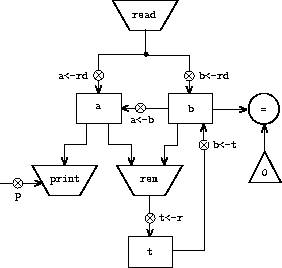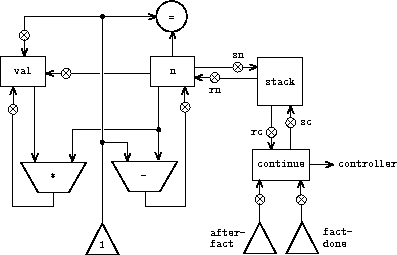# The number of pushes and the maximum stack depth required to compute n!

Measure the number of pushes and the maximum stack depth required to compute `n!` for various small values of `n` using the factorial machine shown in figure 5.11. From your data determine formulas in terms of `n` for the total number of push operations and the maximum stack depth used in computing `n!` for any `n > 1` . Note that each of these is a linear function of `n` and is thus determined by two constants. In order to get the statistics printed, you will have to augment the factorial machine with instructions to initialize the stack and print the statistics. You may want to also modify the machine so that it repeatedly reads a value for `n` , computes the factorial, and prints the result (as we did for the GCD machine in figure 5.4), so that you will not have to repeatedly invoke `get-register-contents` , `set-register-contents!` , and `start` .``````(controller
gcd-loop
test-b
(test (op =) (reg b) (const 0))
(branch (label gcd-done))
(assign t (op rem) (reg a) (reg b))
(assign a (reg b))
(assign b (reg t))
(goto (label test-b))
gcd-done
(perform (op print) (reg a))
(goto (label gcd-loop)))
``````

Figure 5.4: A GCD machine that reads inputs and prints results.``````(controller
(assign continue (label fact-done))     ; set up final return address
fact-loop
(test (op =) (reg n) (const 1))
(branch (label base-case))
;; Set up for the recursive call by saving n and continue.
;; Set up continue so that the computation will continue
;; at after-fact when the subroutine returns.
(save continue)
(save n)
(assign n (op -) (reg n) (const 1))
(assign continue (label after-fact))
(goto (label fact-loop))
after-fact
(restore n)
(restore continue)
(assign val (op *) (reg n) (reg val))   ; val now contains n(n - 1)!
base-case
(assign val (const 1))                  ; base case: 1! = 1